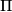IMO Shortlist 2015 problem G8

Kvaliteta:
Avg: 0,0
Težina:
Avg: 9,0

A triangulation of a convex polygon$\Pi$ is a partitioning of$\Pi$ into triangles by diagonals having no common points other than the vertices of the polygon. We say that a triangulation is a Thaiangulation if all triangles in it have the same area.

Prove that any two different Thaiangulations of a convex polygon$\Pi$ differ by exactly two triangles. (In other words, prove that it is possible to replace one pair of triangles in the first Thaiangulation with a different pair of triangles so as to obtain the second Thaiangulation.)

(Bulgaria)

Izvor: https://www.imo-official.org/problems/IMO2015SL.pdf